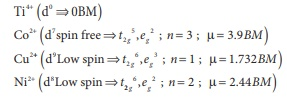# Unit V: Coordination Chemistry - Online Test

Q1. The sum of primary valance and secondary valance of the metal M in the complex [ M ( en)2 ( Ox)] Cl is L
Explaination / Solution:

In the complex [M (en)2 (Ox)] Cl For the central metal ion M3+

The primary valance is = +3

The secondary valance = 6

sum of primary valance and secondary valance = 3+6 = 9

Q2. An excess of silver nitrate is added to 100ml of a 0.01M solution of pentaaqua chloride chromium(III)chloride. The number of moles of AgCl precipitated would be
Explaination / Solution:

The complex is [M(H2O)5 Cl] Cl2

1000 ml of 1M solution of the complex gives 2 moles of Cl ions

1000 ml of 0.01M solution of the complex will give

[100 ml x 0.01M x 2Cl-] / [1000 ml x 1M] = 0.002 moles of C  ions

Q3. A complex has a molecular formula MSO4 Cl. 6H2O .The aqueous solution of it gives white precipitate with Barium chloride solution and no precipitate is obtained when it is treated with silver nitrate solution. If the secondary valence of the metal is six, which one of the following correctly represents the complex?
Explaination / Solution:

Molecular formula: MSO4Cl. 6H2O .

Formation of white precipitate with Barium chloride indicates that SO42- ions are outside  the coordination sphere, and no precipitate with AgNO3 solution indicates that the Cl ions are inside the coordination sphere.Since the coordination number of M is 6, Cl and 5 H2O are ligands, remaining 1 H2O molecular and SO42-  are in the outer coordination sphere.

Q4. Oxidation state of Iron and the charge on the ligand NO in [ Fe ( H2O)5 NO] SO4 are
Explaination / Solution:Q5. As per IUPAC guidelines, the name of the complex [Co( en)2 ( ONO)Cl ] Cl is
Explaination / Solution:
No Explaination.

Q6. IUPAC name of the complex K3 [Al ( C2O4 )3 ] is
Explaination / Solution:
No Explaination.

Q7. A magnetic moment of 1.73BM will be shown by one among the following
Explaination / Solution:Q8. Crystal field stabilization energy for high spin d5 octahedral complex is
Explaination / Solution:

The electronic configuration t2g3, eg2

[3x(-0.4) + 2(0.6)]Δ0

[-1.2 + 1.2]Δ0 =0

Q9. In which of the following coordination entities the magnitude of Δ0 will be maximum?
Explaination / Solution:

In all the complexes, the central metal ion is Co3+, among the given ligands CN is the strongest ligand, which causes large crystal field splitting i.e maximum 0

Q10. Which one of the following will give a pair of enantiomorphs?
Explaination / Solution:

Complexes given in other options (a), (c) and (d) have symmetry elements and hence they are optically inactive.